#### 期刊菜单

Multi-Object Tracking Based on Continuous-Discrete Problem Solving and Group Analysis
DOI: 10.12677/AIRR.2018.73012, PDF, HTML, XML, 下载: 1,093  浏览: 2,265  科研立项经费支持

Abstract: Multi-object tracking technique can improve the tracking performance of a single target by mod-eling the mutual social relationship between different targets, and emergencies groups were quickly detected and predicted scenarios that may occur. Although the existing multi-object tracking technology can balance the data association and trajectory estimation, there are still many problems. This paper introduces the scene information extracted from the background modeling and object recognition to constrained multi-target tracking, and combined into a unified framework for solving with the data association and track estimation of both discrete and continuous classic sub-problems; at the same time, we propose the behavior modeling strategy based on group clustering, whose semantic information places the restriction between adjacent target and its track, which can help improve the trace results. The results of the experiment show that the proposed method is more accurate than the classical multi-target tracking algorithm.

1. 引言

2. 相关研究

3. 算法概述

1) 把多目标跟踪问题看作一个连续-离散的联合求解问题，实现数据关联和轨迹预测两大子问题的同步优化。将数据关联和轨迹预测这两个不同但紧密耦合的问题融合到统一的框架中实现递归式联合求解，并在结合微观社会行为分析的基础上利用标签代价函数对不合理的目标轨迹进行惩罚。

2) 在连续离散联合求解的框架基础上，增加目标组的行为建模，多个近邻的目标之间通常具有相似的位置，走向和速度等动力学特征，从而在一定的时域和空域中构成相对稳定的组。根据一种自底向上的分级聚类策略来测度场景中稳定存在的组，通过对组的外观和运动进行分析将有助于改善目标的数据关联和轨迹估计(图1)。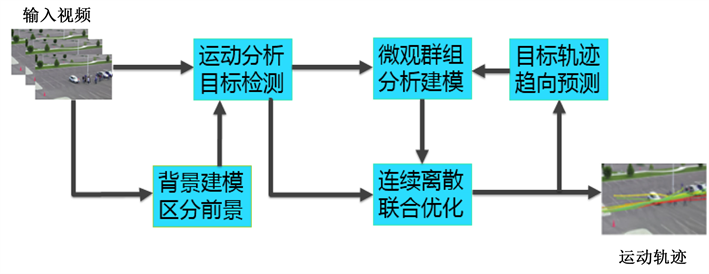Figure 1. The implementation framework of multi-target tracking technology in this paper

4. 连续离散联合求解算法

4.1. 背景建模与运动分析目标检测

4.2. 连续离散问题联合求解

4.3. 连续轨迹模型

${E}_{f}^{te}\left(T\right)=\underset{i=1}{\overset{N}{\sum }}\left({E}_{f}^{te}\left({T}_{i}\right)+\stackrel{^}{{E}_{v}^{te}}\left({T}_{i}\right)\right)$ (1)

${E}_{f}^{te}\left({T}_{i}\right)=\underset{t={s}_{i}}{\overset{{e}_{i}}{\sum }}\underset{j=1}{\overset{|{D}^{t}|}{\sum }}\delta \left[i-{f}_{{d}_{j}^{t}}\right]\cdot {c}_{j}^{t}\cdot {‖{p}_{j}^{t}-{T}_{i}\left(t\right)‖}^{2}$ (2)

$\stackrel{^}{{E}_{v}^{te}}\left({T}_{i}\right)=\underset{\begin{array}{l}{s}_{i}-△\le t<{s}_{i}\\ {e}_{i} (3)

${‖{v}_{i}^{t}-{T}_{i}\left(t\right)‖}^{2}$ 表示通过线性外推得到的虚拟位置V与轨迹上的对应点的欧式距离。Δ表示安全边界，一般取2。三次样条的目的是方便最大限度的能量最小化，在全局封闭的形式下解决最小二乘法问题。

4.4. 离散数据关联

${E}_{T}^{da}\left(f\right)=\underset{d\in D}{\sum }{U}_{d}\left({f}_{d},T\right)+\underset{\left(d,{d}^{\prime }\right)\in E}{\sum }{S}_{d,{d}^{\prime }}\left({f}_{d},{f}_{{d}^{\prime }},{G}_{d},{G}_{{d}^{\prime }}\right)$ (4)

${U}_{{d}_{j}^{t}}\left(l,T\right)={c}_{j}^{t}\cdot {‖{p}_{j}^{t}-{T}_{l}\left(y\right)‖}^{2}$ (5)

${S}_{d,{d}^{\prime }}\left({f}_{d},{f}_{{d}^{\prime }},{G}_{d},{G}_{{d}^{\prime }}\right)=\eta \cdot W\left(d,{d}^{\prime },{G}_{d},{G}_{{d}^{\prime }}\right)\cdot L\left({f}_{d},{f}_{{d}^{\prime }}\right)$ (6)

$\text{ }W\left(d,{d}^{\prime },{G}_{d},{G}_{{d}^{\prime }}\right)=\left\{\begin{array}{l}\mathrm{exp}\left(-\left({‖{v}_{j}^{t}-{v}_{k}^{t+1}‖}^{2}+{‖{o}_{j}^{t}-{o}_{k}^{t+1}‖}^{2}\right)/{\psi }^{2}\right),\text{ }\text{ }\text{ }\text{ }\text{ }\text{ }\text{ }\text{ }\text{ }\text{ }\text{ }\text{ }\text{ }\text{ }\text{ }\text{ }\text{if}\text{\hspace{0.17em}}{G}_{{d}_{j}^{t}}={G}_{{d}_{k}^{t+1}}\\ const,\text{ }\text{ }\text{ }\text{ }\text{ }\text{ }\text{ }\text{ }\text{ }\text{ }\text{ }\text{ }\text{ }\text{ }\text{ }\text{ }\text{ }\text{ }\text{ }\text{ }\text{ }\text{ }\text{ }\text{ }\text{ }\text{ }\text{ }\text{ }\text{ }\text{ }\text{ }\text{ }\text{ }\text{ }\text{ }\text{ }\text{ }\text{ }\text{ }\text{ }\text{ }\text{ }\text{ }\text{ }\text{ }\text{ }\text{ }\text{ }\text{ }\text{ }\text{ }\text{ }\text{ }\text{ }\text{ }\text{ }\text{ }\text{ }\text{ }\text{ }\text{ }\text{ }\text{ }\text{ }\text{ }\text{ }\text{ }\text{ }\text{ }\text{ }\text{ }\text{ }\text{ }\text{ }\text{ }\text{ }\text{ }\text{ }\text{ }\text{ }\text{ }\text{ }\text{ }\text{ }\text{ }\text{ }\text{ }\text{ }\text{ }\text{ }\text{ }\text{ }\text{ }\text{ }\text{ }\text{ }\text{ }\text{ }\text{ }\text{ }\text{ }\text{ }\text{ }\text{ }\text{ }\text{ }\text{ }\text{ }\text{ }\text{ }\text{ }\text{ }\text{ }\text{ }\text{ }\text{ }\text{ }\text{ }\text{ }\text{ }\text{ }\text{ }\text{ }\text{ }\text{ }\text{ }\text{ }\text{ }\text{ }\text{ }\text{ }\text{ }\text{ }\text{ }\text{ }\text{ }\text{ }\text{ }\text{ }\text{ }\text{\hspace{0.17em}}\text{\hspace{0.17em}}\text{ }\text{ }\text{ }\text{ }\text{ }\text{otherwise}\end{array}$ (7)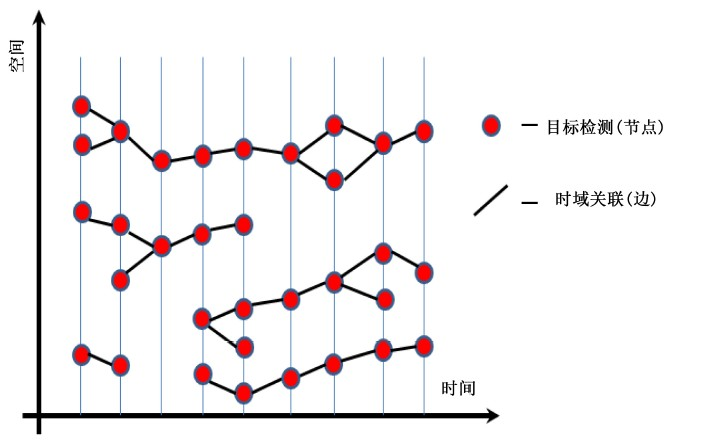Figure 2. Time domain and airspace connection diagram

4.5. 标签成本的离散连续跟踪

$E\left(T,f\right)=\underset{数据项}{\underset{︸}{\underset{d\in D}{\sum }{U}_{d}\left({f}_{d},T\right)}}+\underset{平滑项}{\underset{︸}{\underset{\left(d,{d}^{\prime }\right)\in E}{\sum }{S}_{d,{d}^{\prime }}\left({f}_{d},{f}_{{d}^{\prime }},{g}_{d},{g}_{{d}^{\prime }}\right)}}+\underset{标签项}{\underset{︸}{k\cdot {h}_{f}\left(T\right)}}\text{ }$ (8)

${h}_{f}\left(T\right)=\underset{\begin{array}{l}\text{\hspace{0.17em}}\text{\hspace{0.17em}}\text{\hspace{0.17em}}\text{\hspace{0.17em}}i=1\\ \exists d:{f}_{d}=i\end{array}}{\overset{N}{\sum }}{h}_{i}^{dyn}+{h}_{i}^{per}+{h}_{i}^{fid}+{h}_{i}^{col}+{h}_{i}^{reg}$ (9)

1) ${h}_{i}^{dyn}$ ：由于现实世界中目标速度受物理约束的限制，对速度影响最大的样条曲线的三次系数施加一个惩罚。其定义如式(10)所示。

${h}_{i}^{dyn}=\lambda \cdot \underset{r}{\mathrm{max}}{C}_{i}\left(r,1\right)$ (10)

2) ${h}_{i}^{per}$ ：执行长而且持久的轨迹来避免不必要的ID交换是关键，通过样条曲线，使我们能够确定每一个轨迹的开始和结束点，并对那些起点和终点均远离图像边界的轨迹进行惩罚。如式(11)，其中，和分别代表第条轨迹的起点和终点，而则代表到图像边界的距离运算。

${h}_{i}^{per}=\mu \cdot \left(\stackrel{¯}{b}\left({\tau }_{i}\left({s}_{i}\right)\right)+\stackrel{¯}{b}\left({\tau }_{i}\left({e}_{i}\right)\right)\right)+v\cdot {\left({e}_{i}-{s}_{i}\right)}^{-1}$ (11)

3) ${h}_{i}^{fid}$ ：表示高阶数据保真度，数据项鼓励轨迹附近的检测，以更好地解释所观察到的图像证据，如式(12)，其中，代表满足上述条件的轨迹中所具有的图像帧数。

${h}_{i}^{fid}=\xi \cdot \underset{k}{\sum }{|{G}_{k}|}^{3}$ (12)

4) ${h}_{i}^{col}$ ：表示相互排斥性，如果2个假定的目标位置是彼此接近，加上一个高的罚款，除非至少有一个被标记为离群值在他们最接近的时间，最接近的每个人最小距离的计算和用于定义的标签成本。如式(13)，表示两个轨迹的时间重叠。

${h}_{i}^{col}=\zeta \cdot {\left(\underset{j (13)

5) ${h}_{i}^{reg}$ ：一个固定的正则化成本 ${h}_{i}^{reg}=1$ 用来惩罚大量存在的轨迹。

5. 实验结果与分析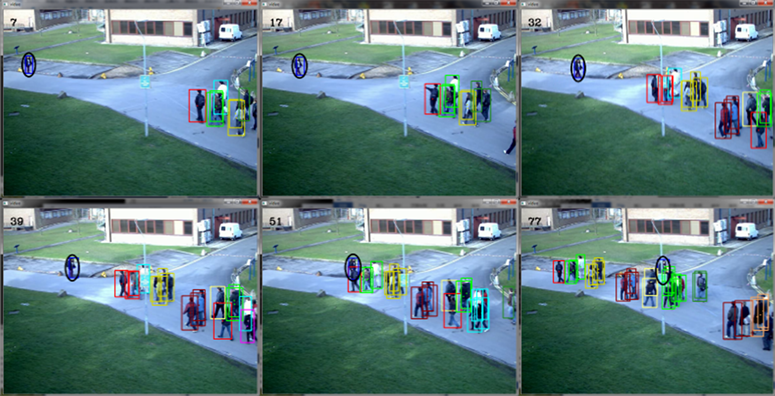Figure 3. Group clustering results for PETS 09 S1L1 dataset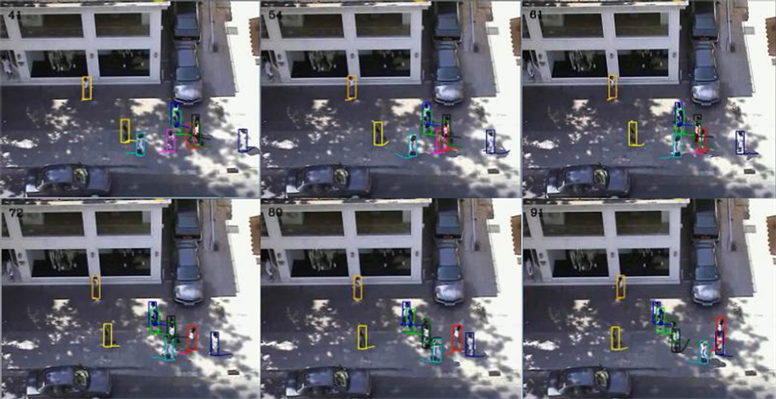Figure 4. Tracking results of the algorithm in the Zara Data Set dataset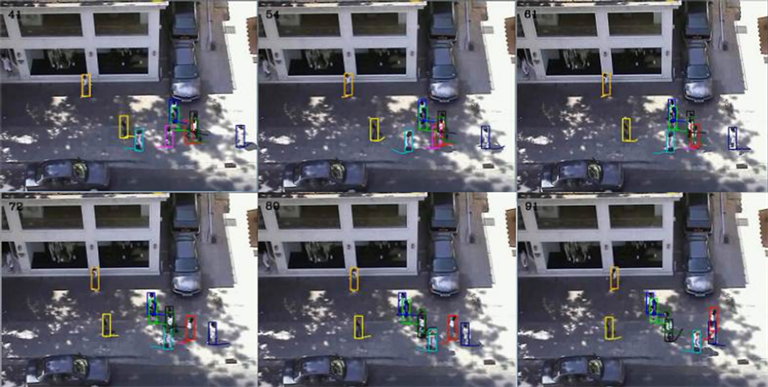Figure 5. Tracking results of our algorithm in the Zara Data Set dataset

6. 结束语

  Reid, D.B. (1979) An Algorithm for Tracking Multiple Targets. IEEE Transaction on Automatics Control, 1202-1211.  Breitenstein, M.D., Reichlin, F., Leibe, B., et al. (2011) Online Multiperson Tracking-by-Detection from a Single, Uncalibrated Camera. IEEE Transactions on Pattern Analysis & Machine Intelligence, 33, 1820. https://doi.org/10.1109/TPAMI.2010.232  Jiang, H., Fels, S. and Little, J.J. (2007) A Linear Programming Approach for Multiple Object Tracking. IEEE Conference on Computer Vision and Pattern Recognition, 1-8.  Kunz, T.H. and Betke, M. (2011) Efficient Track Linking Methods for Track Graphs Using Network-Flow and Set-Cover Techniques. IEEE Conference on Computer Vision and Pattern Recognition, Colorado Springs, CO, 20-25 June 2011, 1185-1192.  Leibe, B., Cornelis, N., Cornelis, K., et al. (2007) Dynamic 3D Scene Analysis from a Moving Vehicle. IEEE Conference on Computer Vision and Pattern Recognition, CVPR’07, 2, 1308-1313.  Andriyenko, A. and Schindler, K. (2010) Globally Optimal Multi-target Tracking on a Hexagonal Lattice. Computer Vision-ECCV 2010, European Conference on Computer Vision, Heraklion, Crete, 5-11 September 2010, 466-479.  Andriyenko, A. and Schindler, K. (2016) Multi-Target Tracking by Continuous Energy Minimization. IEEE Transac-tions on Pattern Analysis & Machine Intelligence, 38, 2054. https://doi.org/10.1109/TPAMI.2015.2505309  Dicle, C., Camps, O.I. and Sznaier, M. (2013) The Way They Move: Tracking Multiple Targets with Similar Appearance. IEEE International Conference on Computer Vision, Sydney, NSW, 1-8 December 2013, 2304-2311.  Pauwels, K., Rubio, L. and Ros, E. (2014) Real-Time Model-Based Articulated Object Pose Detection and Tracking with Variable Rigidity Constraints. IEEE Conference on Computer Vision and Pattern Recognition, Columbus, OH, 23-28 June 2014, 3994-4001.  Sun, L., Ai, H. and Lao, S. (2014) Activity Group Localization by Modeling the Relations among Participants. Computer Vision-ECCV 2014, 8689, 741-755.  Ciptadi, A. and Rehg, J.M. (2015) Minimizing Human Effort in Interactive Tracking by Incremental Learning of Model Parameters. IEEE International Conference on Computer Vision, Santiago, 7-13 December 2015, 4382-4390.  Zhu, F., Wang, X. and Yu, N. (2014) Crowd Tracking with Dynamic Evolution of Group Structures. Computer Vision-ECCV 2014, 8694, 139-154.  Shao, J., Loy, C.C. and Wang, X. (2014) Scene-Independent Group Profiling in Crowd. Computer Vision and Pattern Recognition, Columbus, OH, 23-28 June 2014, 2227-2234.  Nebehay, G. and Pflugfelder, R. (2015) Clustering of Static-Adaptive Correspondences for Deformable Object Tracking. Computer Vision and Pattern Recognition, Boston, MA, 7-12 June 2015, 2784-2791.  Chen, X., Qin, Z., An, L., et al. (2014) An Online Learned Elementary Grouping Model for Multi-target Tracking. IEEE Conference on Computer Vision and Pattern Recognition, Columbus, OH, 23-28 June 2014, 1242-1249.  余婷婷. 浅论混合高斯背景建模方法[J]. 黑龙江科技信息, 2012(15): 60.  Roth, S. (2012) Discrete-Continuous Optimization for Multi-Target Tracking. Computer Vision and Pattern Recognition, Providence, RI, 16-21 June 2012, 1926-1933.